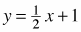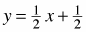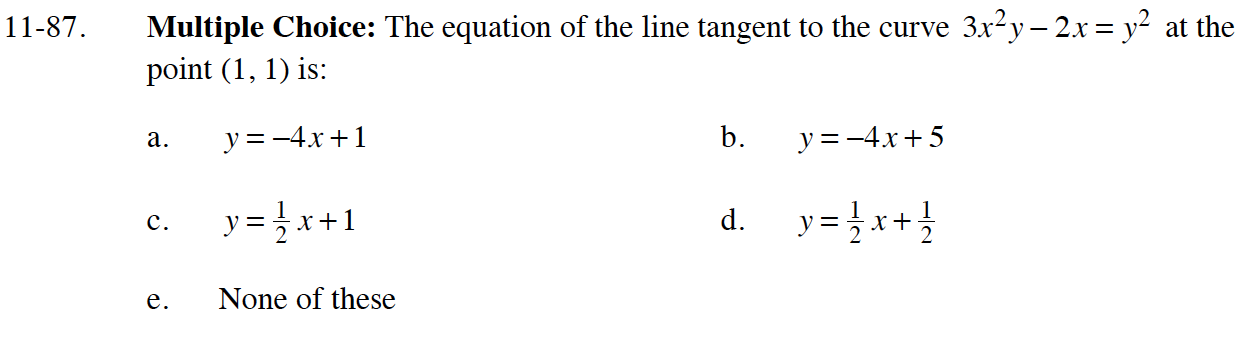### Home > CALC > Chapter 11 > Lesson 11.2.4 > Problem11-87

11-87.
1. Multiple Choice: The equation of the line tangent to the curve 3x2 y − 2x = y2 at the point (1, 1) is: Homework Help ✎

1. y = −4x + 1

2. y = −4x + 5

3.4.5. None of these$6xy+3x^2y^\prime-2=2yy^\prime$

$6(1)(1)+3(1)^2y^\prime-2=2(1)y^\prime$

Solve for y′. This is the slope of the line.